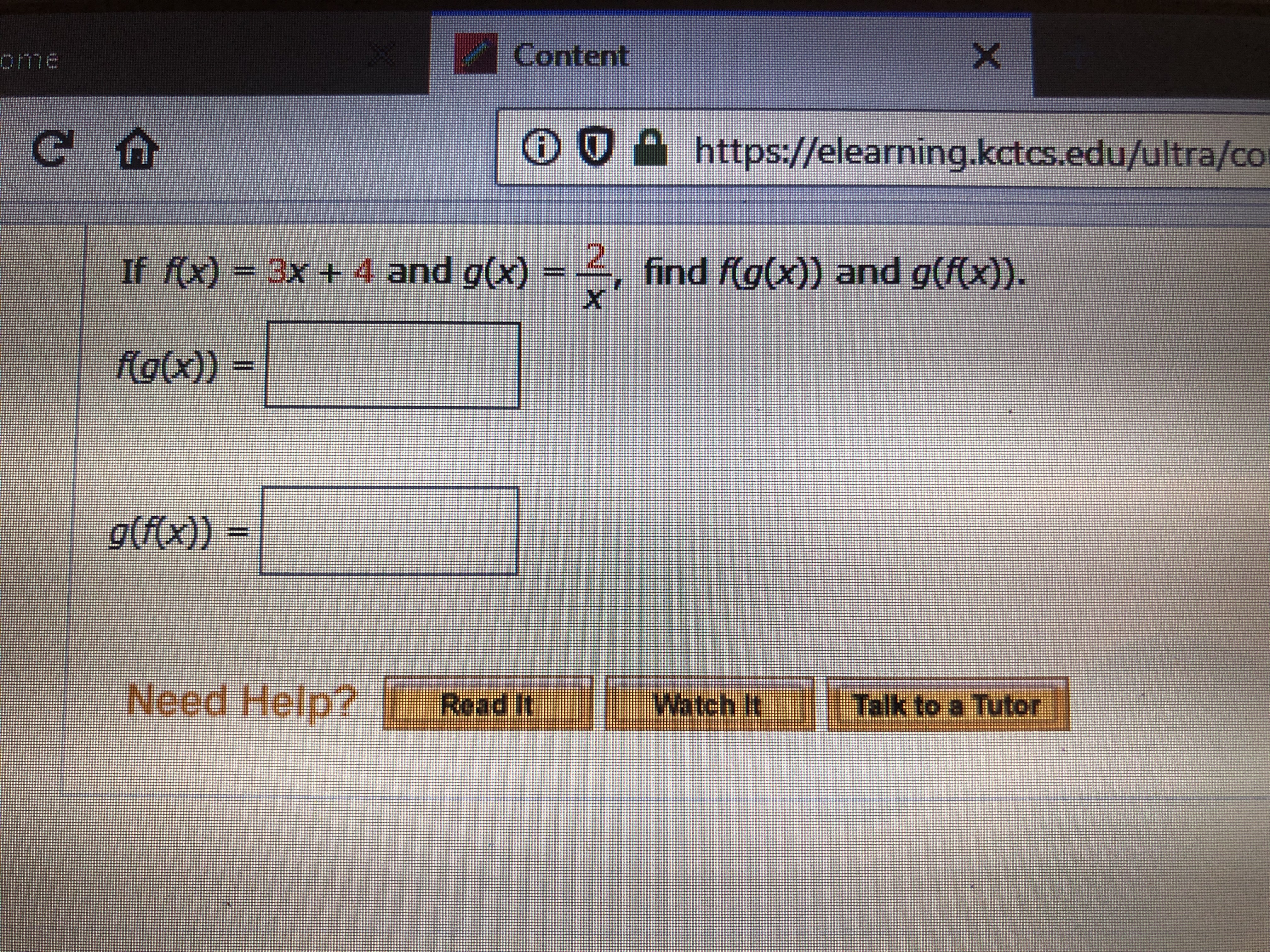# ContentXomeChttps://elearning.kctcs.edu/ultra/coIf f(x)3x + 4 and g(x)find f(g(x)) and g(f(x)).XfG(X))- ((C))0Watch It Talk to a Tutor

Question

what are the blankshelp_outlineImage TranscriptioncloseContent X ome C https://elearning.kctcs.edu/ultra/co If f(x) 3x + 4 and g(x) find f(g(x)) and g(f(x)). X fG(X)) - ((C))0 Watch It Talk to a Tutor fullscreen
check_circleExpert Solution
Step 1

To find the expressions (formulae) for the composites of the given functions fx) and g(x)

Step 2

To find f(g(x)) , first find g(x) and substitute g(x) in the formula for f(x)

Step 3

To find g(f(x)) ,  substitute f(x...

### Want to see the full answer?

See Solution

#### Want to see this answer and more?

Solutions are written by subject experts who are available 24/7. Questions are typically answered within 1 hour*

See Solution
*Response times may vary by subject and question
Tagged in

### Algebra